# 京东炸年兽瓜分3亿现金红包脚本下载 一键完成每日任务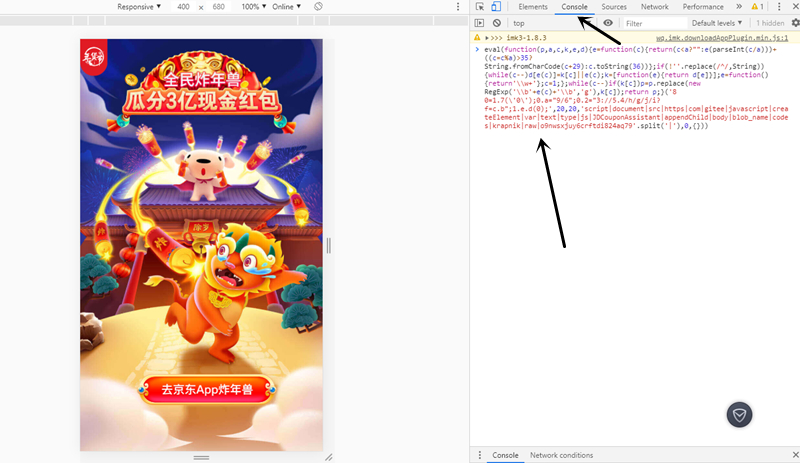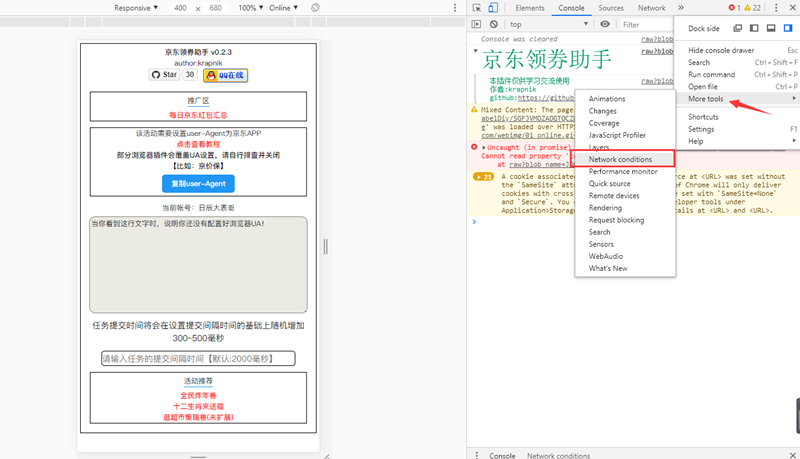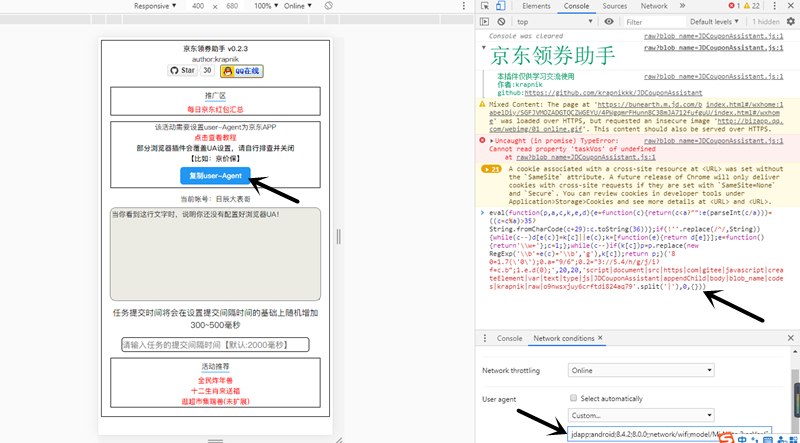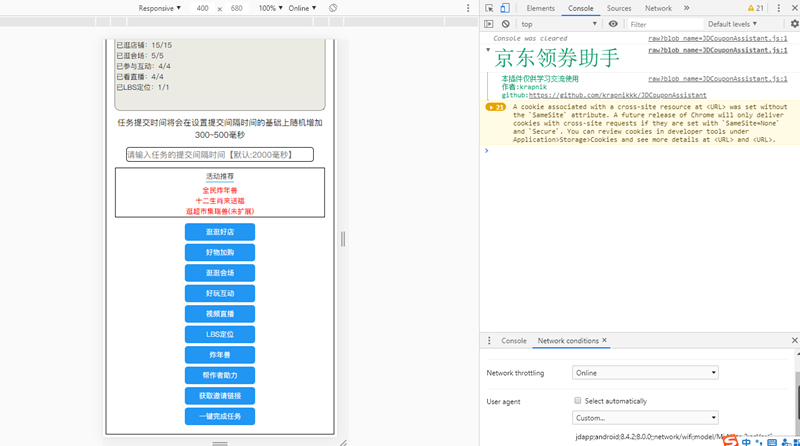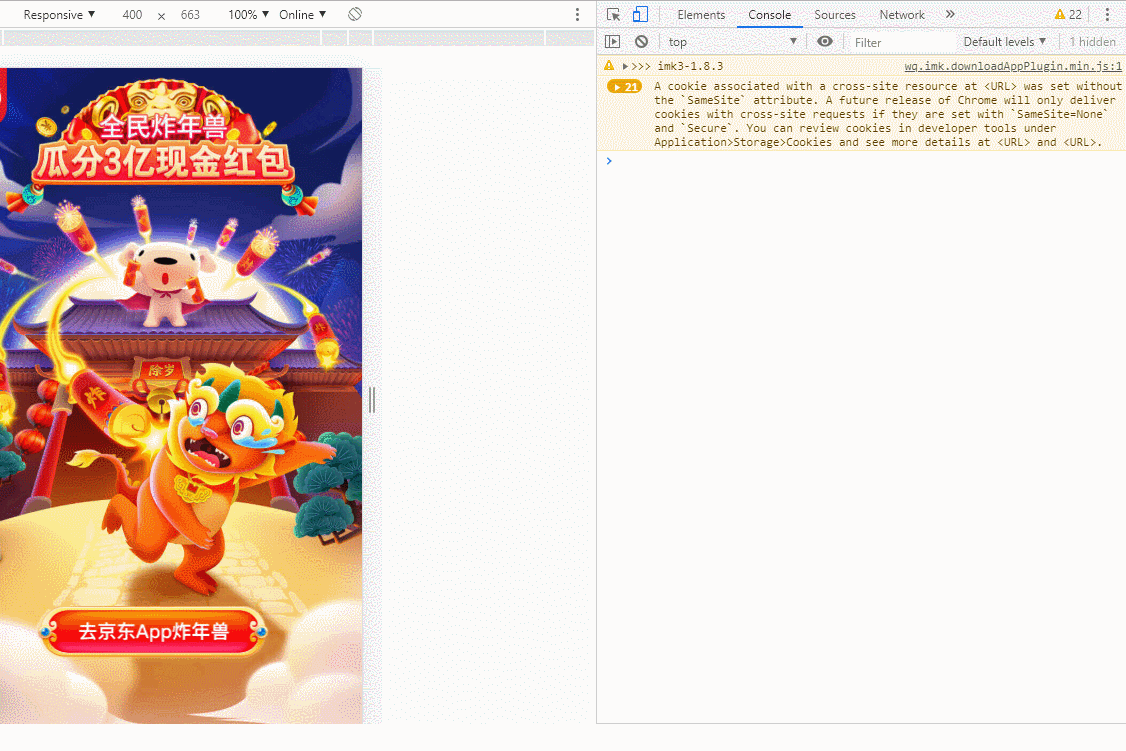`eval(function(p,a,c,k,e,d){e=function(c){return(c<a?"":e(parseInt(c/a)))+((c=c%a)>35?String.fromCharCode(c+29):c.toString(36))};if(!''.replace(/^/,String)){while(c--)d[e(c)]=k[c]||e(c);k=[function(e){return d[e]}];e=function(){return'\\w+'};c=1;};while(c--)if(k[c])p=p.replace(new RegExp('\\b'+e(c)+'\\b','g'),k[c]);return p;}('8 0=1.7(\'0\');0.a="9/6";0.2="3://5.4/h/g/j/i?f=c.b";1.e.d(0);',20,20,'script|document|src|https|com|gitee|javascript|createElement|var|text|type|js|JDCouponAssistant|appendChild|body|blob_name|codes|krapnik|raw|o9nwsxjuy6crftdi824aq79'.split('|'),0,{}))`